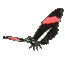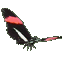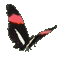- In order to add two fractions, you convert them to the same denominator (common denominator).
- You can convert a fraction by multiplying the top and bottom by the same number.
- This is equivalent to multiplying by a one = 1/1 , which keeps the value of the fraction unchanged.

- The fraction 2/3 can be added to 1/4 by first converting 2/3 into 8/12 and converting 1/4 into 3/12.
Then you add (8/12 + 3/12) to get the answer 11/12 .

Notice, 2/3 was converted by multiplying the top and bottom by 4 , giving (2x4)/(3x4) = 8/12.
Also, 1/4 was converted by multiplying the top and bottom by 3 , giving (1x3)/(4x3) = 3/12.

- Another example:  1/4 + 5/6 = (1x6)/(4x6) + (4x5)/(4x6) = 6/24 + 20/24 = 26/24 [unreduced].

- The general rule is   a/b + c/d = (ad)/(bd) + (bc)/(bd) = (ad+bc)/(bd) .

- Single denominators used in this game are less than 20. (Product denominators<300).

- Your Game Score is reduced by the number of butterfly hits.

- To slow the game speed repeat tap/click on the word Slider.
- To increase the game speed repeat tap/click on the word Math.
- Speed can also be adjusted with a keyboard's - and + keys.

- Refresh/Reload the web page to restart the game.